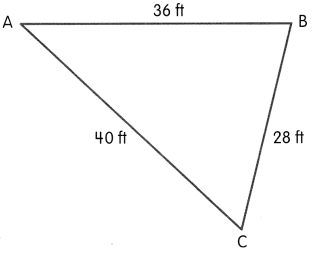# Math in Focus Grade 2 Cumulative Review for Chapters 13 and 14 Answer Key

This handy Math in Focus Grade 2 Workbook Answer Key Cumulative Review for Chapters 13 and 14 detailed solutions for the textbook questions.

## Math in Focus Grade 2 Cumulative Review for Chapters 13 and 14 Answer Key

Concepts and Skills

Fill in the blanks.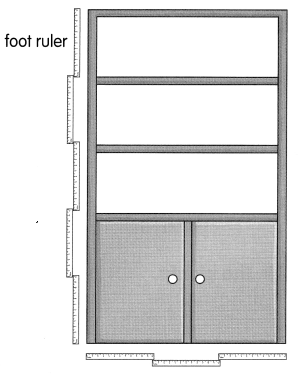Question 1.
The bookshelf ¡s _________ feet tall.

The bookshelf ¡s 5 feet tall.

Question 2.
The bookshelf is _________ feet wide.

The bookshelf is 3 feet wide.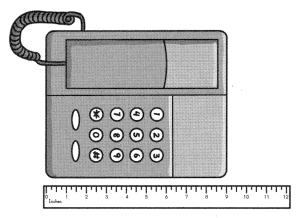Question 3.
The telephone is __________ inches long.

The telephone is 9 inches long.

Fill in the blanks with feet or inches.

Question 4.
A car is about 8 _________ long.

A car is about 8 feet long.

Question 5.
The grip on a bicycle handle bar is about 6 __________ long.

The grip on a bicycle handle bar is about 6 inches long.

Look at each drawing. Then fill in the blanks.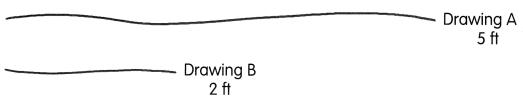Question 6.
Which drawing is shorter?

Drawing B is shorter.

Question 7.
How much shorter?
__________ feet

5 – 2 = 3

Drawing B is 3 feet shorter.

Fill in the blanks.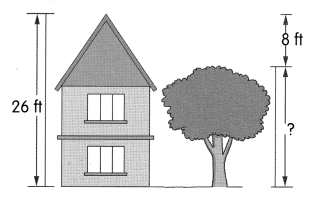Question 8.
The tree is about ___________ feet shorter than the house.

The tree is about 8 feet shorter than the house.

Question 9.
The tree is about ___________ feet tall.

The tree is about 18 feet tall. ( 26 – 8 = 18 )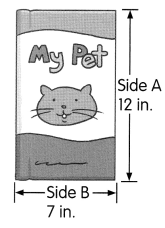Question 10.
Side A is _________ inches longer than Side B.

Side A is 5 inches longer than Side B.  ( 12 – 7 = 5 )

Question 11.
The total length of the two sides is ___________

The total length of the two sides is 19 ( 12 + 7 ) inches.

Use the picture to answer each question.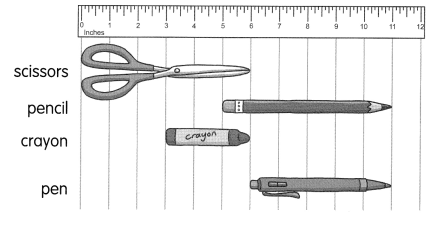Question 12.
What is the length of the pen?

length of the pen is 5 inches. ( 11 – 6 = 5 )

Question 13.
Which is longer, the scissors or the pen?

Scissor is long ( 6 > 5 )

Question 14.
The shortest item is the __________.

The shortest item is the crayon ( 3 inches ).

Question 15.
Which two items have the same length?

Scissor and pencil have the same length ( 6 inches ).

Question 16.
The total length of 2 pens and 3 crayons is __________ inches.

The length of one pen is 5 inches

The length of one crayon is 3 inches

So, 2 x 5 + 3 x 3

10 + 9 = 19

Therefore, The total length of 2 pens and 3 crayons is 19 inches.

Question 17.
The length of __________ crayons is more than the length of 2 pens.

4 x 3 > 2 x 5

12 > 10

Therefore, The length of 4 crayons is more than the length of 2 pens.

Draw the hands to show the time. Then fill in the blanks.

Question 18.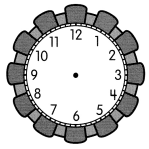The time is 4:15.
It is 15 minutes after _________ o’clock.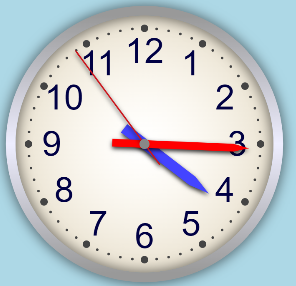It is 15 minutes after 4 o’clock.

Question 19.The time is 9:55.
It is _________ minutes after 9 o’clock.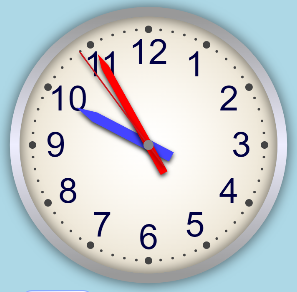It is 55 minutes after 9 o’clock.

Question 20.The time is 6:45.
It is _________ minutes after 6 o’clock.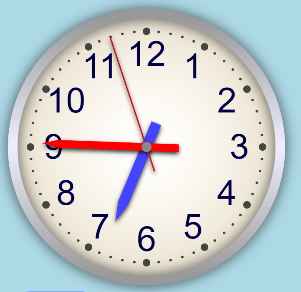It is 45 minutes after 6 o’clock.

Question 21.The time is 11:15.
It is 15 minutes after _________ o’clock.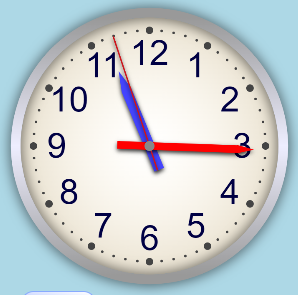It is 15 minutes after 11 o’clock.

Match. Then write the time in words under each digital clock.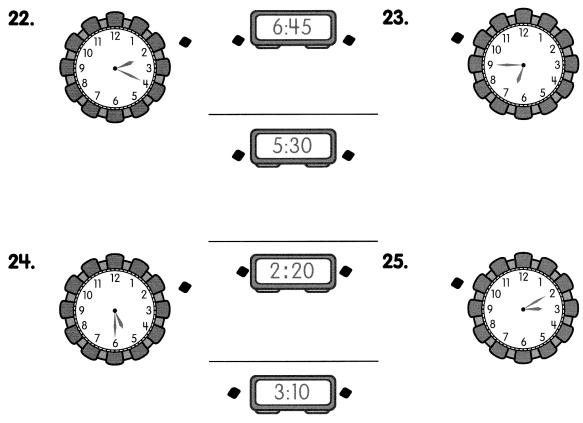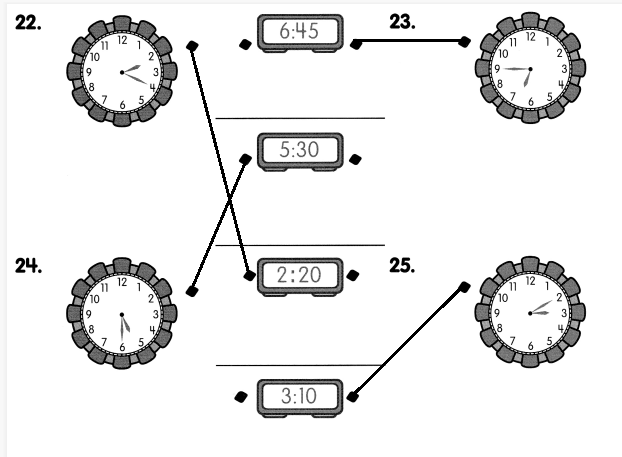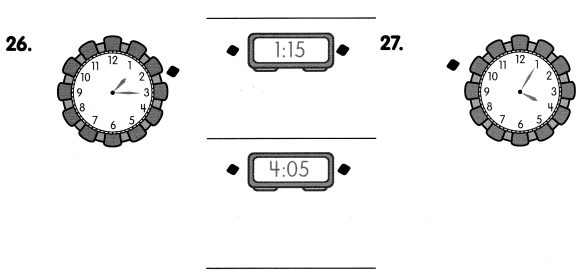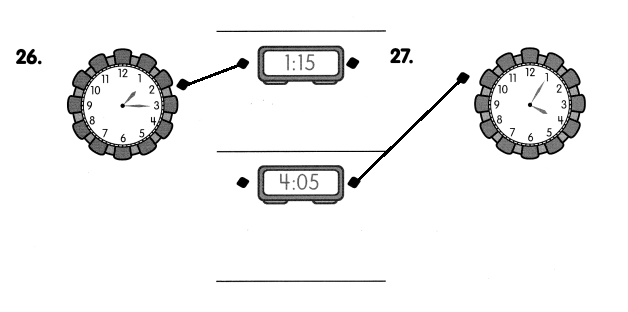Fill in the blanks with A.M. or P.M.

Question 28.
On Saturdays, Marjorie usually wakes up at 8:00 __________________

On Saturdays, Marjorie usually wakes up at 8:00 AM

Question 29.
She has lunch at her grandparents’ house at 12:30 __________________

She has lunch at her grandparents’ house at 12:30 PM

Question 30.
She helps her mother do house chores at 9:30 __________________

She helps her mother do house chores at 9:30 AM

Question 31.
She plays board games with her grandpa at 3:00 __________________

She plays board games with her grandpa at 3:00 PM

Question 32.
She goes home at 5:00 __________________

She goes home at 5:00 PM

Now list the times in Exercises 28 to 32 in order from the beginning of the day.

Question 33.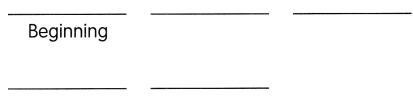Fill in the blanks with the time. Check your answer by drawing the hands on the clock.

Question 34.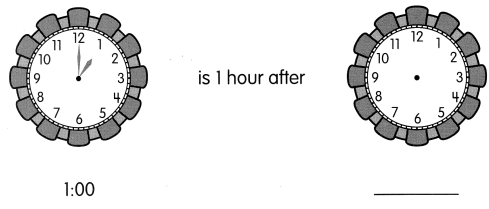Question 35.Question 36.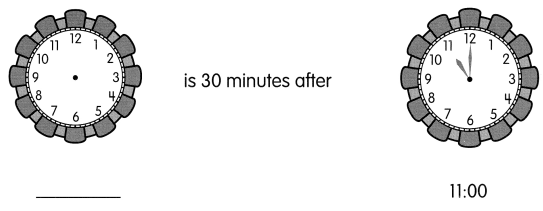Question 37.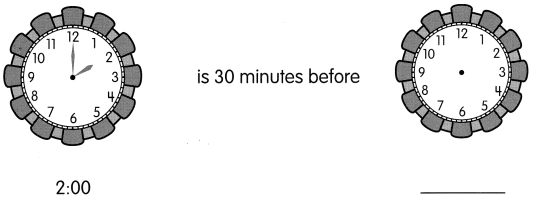Fill in the blanks with before or after.

Question 38.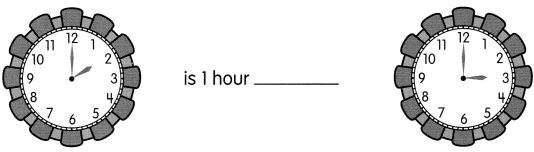Question 39.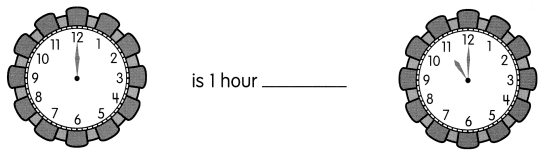Question 40.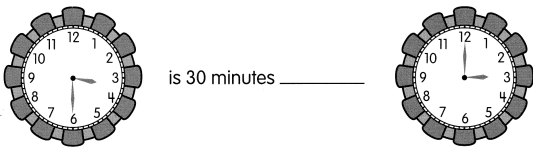Question 41.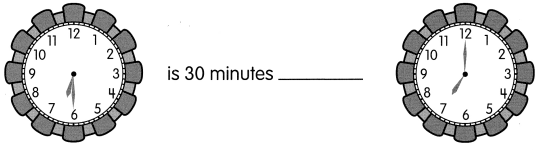Problem Solving

Question 42.
Ben uses a piece of rope to form a triangle.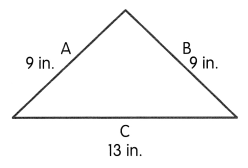a. What is the length of the rope?
The rope is __________ inches long.

b. How much longer is the sum of the two shorter sides than the longest side of the triangle?
The sum of the two shorter sides is ___________ inches longer than the longest side.

Question 43.
Rope A is 56 feet long. Rope B is 47 feet longer than Rope A. Rope C is 71 feet shorter than Rope B.
a. How long is Rope B?
Rope B is _________ feet long.

b. How long is Rope C?
Rope C is ________ feet long.

Question 44.
Jason is 71 inches tall. Rodney is 12 inches shorter than Jason. Marco is 18 inches taller than Rodney.
a. How tall is Rodney?
Rodney is _________ inches tall.

b. How tall is Marco?
Marco is _________ inches tall.